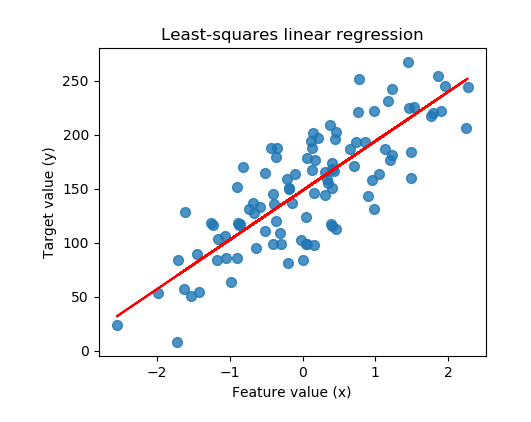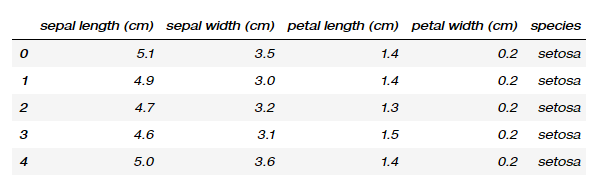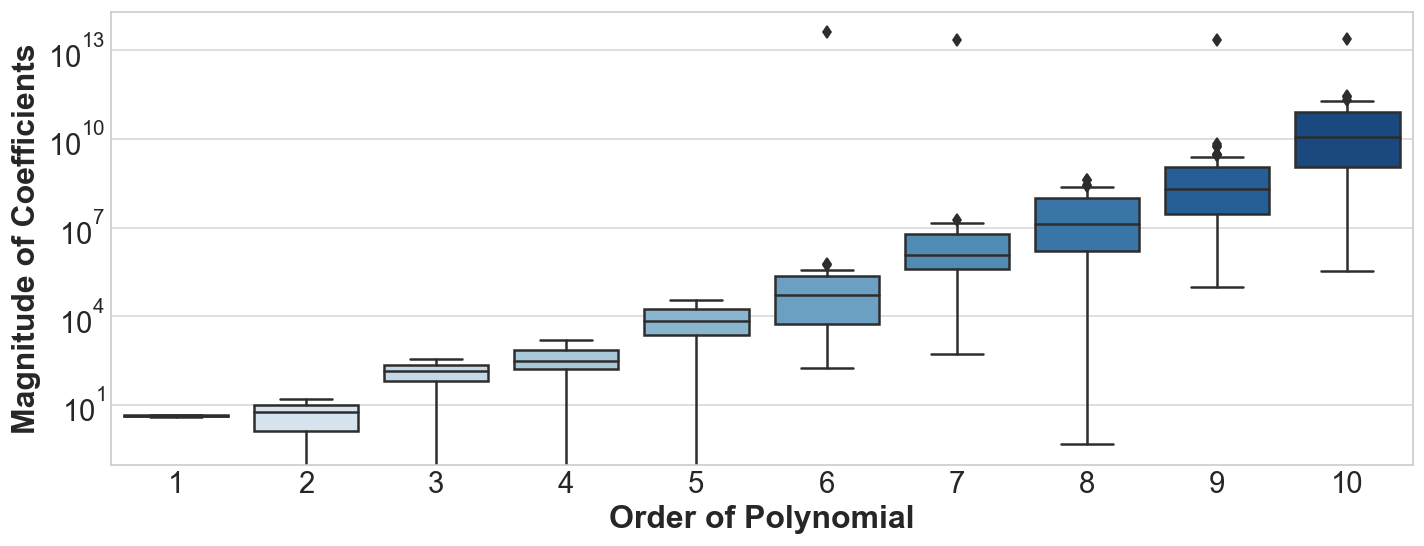1597903560

# Linear regressions with scikit-learn

## The ConceptSimple linear regression

Briefly, linear regressions are about finding a best fit linear line (usually judged by the R squared metric) through a set of data points. This line would achieve a better fit through minimizing the differences (residuals) between the actual and predicted Y data points for a given X data point. With an example below, the blue points represent the actual Y values, while the red line represents the vector of predicted Y values.

Excitingly, simple linear regressions are one of the easiest forms of supervised machine learning!

## The Data

To begin with, I’ll be using the iris dataset which is quite a widely covered dataset in the data space and can be easily found online. It contains a set of 150 records under 5 attributes — Petal Length, Petal Width, Sepal Length, Sepal width and Class(Species).Quick peek at the data set

## The Packages

1. sklearn modules (train_test_split , LinearRegression, make_regression, load_iris) — These will be necessary in loading the iris dataset, preparation of data and fitting of the model.
2. _matplotlib pyploy _module — Needed to plot the results.
3. _pandas _and numpy packages — Needed to manipulate the dataframe and its constituent values

#data-science #machine-learning #python #data-analytics #data-analysis #data science

## Buddha Community1618278600

## Scikit-Learn Is Still Rocking, Been Introduced To French President

Amilestone for open source projects — French President Emmanuel Macron has recently been introduced to Scikit-learn. In fact, in a recent tweet, Scikit-learn creator and Inria tenured research director, Gael Varoquaux announced the presentation of Scikit-Learn, with applications of machine learning in digital health, to the president of France.

He stated the advancement of this free software machine learning library — “started from the grassroots, built by a community, we are powering digital revolutions, adding transparency and independence.”

#news #application of scikit learn for machine learning #applications of scikit learn for digital health #scikit learn #scikit learn introduced to french president1598352300

## Regression: Linear Regression

Machine learning algorithms are not your regular algorithms that we may be used to because they are often described by a combination of some complex statistics and mathematics. Since it is very important to understand the background of any algorithm you want to implement, this could pose a challenge to people with a non-mathematical background as the maths can sap your motivation by slowing you down.In this article, we would be discussing linear and logistic regression and some regression techniques assuming we all have heard or even learnt about the Linear model in Mathematics class at high school. Hopefully, at the end of the article, the concept would be clearer.

**Regression Analysis **is a statistical process for estimating the relationships between the dependent variables (say Y) and one or more independent variables or predictors (X). It explains the changes in the dependent variables with respect to changes in select predictors. Some major uses for regression analysis are in determining the strength of predictors, forecasting an effect, and trend forecasting. It finds the significant relationship between variables and the impact of predictors on dependent variables. In regression, we fit a curve/line (regression/best fit line) to the data points, such that the differences between the distances of data points from the curve/line are minimized.

#regression #machine-learning #beginner #logistic-regression #linear-regression #deep learning1594271340

## A Deep Dive into Linear Regression

Let’s begin our journey with the truth — machines never learn. What a typical machine learning algorithm does is find a mathematical equation that, when applied to a given set of training data, produces a prediction that is very close to the actual output.

Why is this not learning? Because if you change the training data or environment even slightly, the algorithm will go haywire! Not how learning works in humans. If you learned to play a video game by looking straight at the screen, you would still be a good player if the screen is slightly tilted by someone, which would not be the case in ML algorithms.

However, most of the algorithms are so complex and intimidating that it gives our mere human intelligence the feel of actual learning, effectively hiding the underlying math within. There goes a dictum that if you can implement the algorithm, you know the algorithm. This saying is lost in the dense jungle of libraries and inbuilt modules which programming languages provide, reducing us to regular programmers calling an API and strengthening further this notion of a black box. Our quest will be to unravel the mysteries of this so-called ‘black box’ which magically produces accurate predictions, detects objects, diagnoses diseases and claims to surpass human intelligence one day.

We will start with one of the not-so-complex and easy to visualize algorithm in the ML paradigm — Linear Regression. The article is divided into the following sections:

1. Need for Linear Regression

2. Visualizing Linear Regression

3. Deriving the formula for weight matrix W

4. Using the formula and performing linear regression on a real world data set

Note: Knowledge on Linear Algebra, a little bit of Calculus and Matrices are a prerequisite to understanding this article

Also, a basic understanding of python, NumPy, and Matplotlib are a must.

## 1) Need for Linear regression

Regression means predicting a real valued number from a given set of input variables. Eg. Predicting temperature based on month of the year, humidity, altitude above sea level, etc. Linear Regression would therefore mean predicting a real valued number that follows a linear trend. Linear regression is the first line of attack to discover correlations in our data.

Now, the first thing that comes to our mind when we hear the word linear is, a line.

Yes! In linear regression, we try to fit a line that best generalizes all the data points in the data set. By generalizing, we mean we try to fit a line that passes very close to all the data points.

But how do we ensure that this happens? To understand this, let’s visualize a 1-D Linear Regression. This is also called as Simple Linear Regression

#calculus #machine-learning #linear-regression-math #linear-regression #linear-regression-python #python1596458460

## Ridge Regression: Regularization Fundamentals

Regularization is a method used to reduce the variance of a Machine Learning model; in other words, it is used to reduce overfitting. Overfitting occurs when a machine learning model performs well on the training examples but fails to yield accurate predictions for data that it has not been trained on.

In theory, there are 2 major ways to build a machine learning model with the ability to generalize well on unseen data:

1. Train the simplest model possible for our purpose(according to Occam’s Razor).
2. Train a complex or more expressive model on the data and perform regularization.

It has been observed that method #2 yields the best performing models by contemporary standards. In other words, we want our model to have the ability to capture highly complex functions. However, to overcome overfitting, we regularize it.

# Objective:

In the present article we will discuss:

1. Effect of regularization on coefficients and model performance.
2. Data pre-processing steps mandatory for regularization.

We will use the Boston Housing Prices Data available in scikit-learn.

# Data

``````import pandas as pd
import numpy as np
import matplotlib.pyplot as plt
from sklearn import preprocessing, linear_model, model_selection, metrics, datasets, base
# Load LSTAT and RM Features from Boston Housing Data
X = pd.DataFrame(bos.data, columns = bos.feature_names)[['LSTAT', 'RM']]
y = bos.target
``````

# Effect of regularization on Model Coefficients

Regularization penalizes a model for being more complex; for linear models, it means regularization forces model coefficients to be smaller in magnitude.

First let us understand the problems of having large model coefficients. Let us assume a linear model trained on the above data. Let us assume the regression coefficient for the input LSTAT to be large. Now, this means, that assuming all the features are scaled, for a very small change in LSTAT, the prediction will change by a large amount. This simply follows from the Equation for Linear Regression.

In general, inputs having significantly large coefficients tend to drive the model predictions when all the features take values in similar ranges. This becomes a problem if the important feature is noisy or the model overfits to the data — because this causes the model predictions to be either driven by noise or by insignificant variations in LSTAT.

In other words, in general, we want the model to to have coefficients of smaller magnitudes.

Let us See if regularizing indeed reduces the magnitude of coefficients. To visualize this, we will generate polynomial features from our data of all orders from 1 to 10 and make a box-plot of the magnitude of coefficients of the features for:

1. Un-regularized Linear Regression
2. L2 Regularized Linear Regression(Ridge)

Note: Before fitting the model, we are standardizing the inputs.

``````model = linear_model.LinearRegression()
scaler = preprocessing.StandardScaler().fit(X_train)
X_scaled = scaler.transform(X_train)
model.fit(X_scaled , y_train)
coefs = pd.DataFrame()
coefs['Features'] = X.columns
coefs['1'] = np.abs(model.coef_)
for order in range(2, 11):
poly = preprocessing.PolynomialFeatures(order).fit(X_train)
X_poly = poly.transform(X_train)
scaler = preprocessing.StandardScaler().fit(X_poly)
model = linear_model.LinearRegression().fit(scaler.transform(X_poly), y_train)
coefs = pd.concat([coefs, pd.Series(np.abs(model.coef_), name = str(order))], axis = 1)

sns.boxplot(data = pd.melt(coefs.drop('Features', axis = 1)), x = 'variable', y = 'value',
order = [str(i) for i in range(1, 11)], palette = 'Blues')
ax = plt.gca()
ax.yaxis.grid(True, alpha = .3, color = 'grey')
ax.xaxis.grid(False)
plt.yscale('log')
plt.xlabel('Order of Polynomial', weight = 'bold')
plt.ylabel('Magnitude of Coefficients', weight = 'bold')
``````Distribution of Linear Model(Not Regularized) Coefficients for polynomials of various degrees

We observe the following:

1. As the order of polynomial increases, the linear model coefficients become more likely to take on large values.
2. The largest coefficient of the 10th order polynomial is over 10¹² times the magnitude of the largest coefficient of the first order features.
3. Most of the higher order polynomials have coefficients in the order of 10⁴ to 10¹⁰

Let us now, perform the same exercise with Ridge(L2 Regularized) Regression.

#regression #linear-regression #scikit-learn #regularization #ridge-regression #deep learning1590402180

## Machine Learning 101 : Regression

In the last article we talked about Classification, which outputs a category based on the training data. But what if we want our output to be numerical ? For example, predict property prices in a certain area, classification won’t help us in these kind of problems. Therefore, let’s take a quick look at Linear Regression and it’s inner mechanics.

#machine-learning #scikit-learn #linear-regression #regression